GMAT Question of the Day - Daily to your Mailbox; hard ones only

 It is currently 18 Nov 2019, 21:55### GMAT Club Daily Prep

#### Thank you for using the timer - this advanced tool can estimate your performance and suggest more practice questions. We have subscribed you to Daily Prep Questions via email.

Customized
for You

we will pick new questions that match your level based on your Timer History

Track

every week, we’ll send you an estimated GMAT score based on your performance

Practice
Pays

we will pick new questions that match your level based on your Timer History

#### Not interested in getting valuable practice questions and articles delivered to your email? No problem, unsubscribe here.# Tips and Tricks for Absolute Values

Author Message
TAGS:

### Hide Tags

Current StudentV
Joined: 19 Mar 2012
Posts: 4273
Location: India
GMAT 1: 760 Q50 V42GPA: 3.8
WE: Marketing (Non-Profit and Government)
Tips and Tricks for Absolute Values  [#permalink]

### Show Tags

GMAT Tip of the Week: Tips and Tricks for Absolute Values

This topic is a part of the GMAT Club Tip of the Week Series

To score beyond Q49 on GMAT, it is very important that you master Algebra as it has significant application in other sections such as Number Properties, Word Problems and Geometry. In this article, we would help learn you a simple yet effective approach to solve questions on one of the most confusing topics in Algebra - Absolute Value equations. Then we share a link with you to learn Absolute Inequalities, so that you can solve any Absolute value question with ease.

We suggest that you follow the sequence mentioned below:

1. Get started by going through the video lessons embedded above to learn the basics.
2. Then go through the following questions in this article to learn how to solve Absolute Equation questions that involve multiple modulus.
3. Finally go through the concept of Absolute Inequalities, to continue learning.

Example 1

If x is an integer, then find the values of x that satisfy the equation ||x + 9| - 15| = 10?

Solution

As we can see that we are given one modulus inside another modulus:

o That is, |x+9| is inside ||x+9|-15|.
o And, we are asked to find out all the possible values of x such that ||x + 9| - 15| = 10.

So, how do we solve this question?

o Well, we will simply apply the basic properties of absolute values.

1. We will first solve the outermost modulus.

a. And, while solving it, we can substitute the inner modulus with an appropriate variable such as y.
2. Once we have solved the outer modulus, we will get the values for the assumed variable y and then we can simplify the inner modulus, by substituting back the modulus.
3. Now, this process will continue even if we had more than 2 moduli in the question.
a. We will first solve the outermost and then we move from outermost modulus to the inner moduli.

So, let's apply the same process here.

o We will start from the outer modulus.
o Therefore, let us substitute the inner modulus, |x+9|= y.
 Hence, we get |y - 15| = 10.

o Now, let's focus our energy on solving the equation |y - 15| = 10
 From the concept video, we learnt that the form |x - a| = b represents numbers on the number line that are at a distance of 'b' units from 'a'
 Applying this logic, to the equation |y - 15| = 10, we can say that y is a number at a distance of 10 units from "15".

o So, let's find out all the numbers, which are at a distance of 10 units from the point '15', by representing it on a number line.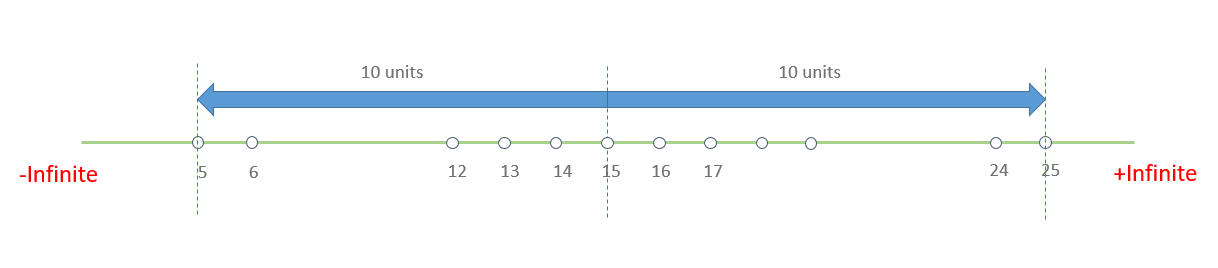o If we observe the diagram above, we can notice that there can be two possible numbers, which are at a distance of 10 units from 15.
 One on the left side of 15, which is 10 units away from it = 15 - 10 = 5 and
 One on the right side of 15, which is 10 units away from it = 15 + 10 = 25

o Hence, the possible values of y are 5 and 25

o Substituting back the modulus in the above equation, we get,
 |x + 9| = 5, or
 |x + 9| = 25

o Let's solve each modulus individually and find the values of x

o Considering the first case, where |x + 9| = 5, we need to find the values of x, which are at a distance of 5 units from -9.
 Now, most of you might wonder, why did we consider -9, instead of 9. But, if we observe the general equation of modulus carefully, it is of the form |x - a| = b., where x is b units away from point 'a'.
 And, in this case, we can write |x + 9| = 5 as |x - (-9) | = 5.

Note: So, remember to notice the sign of 'a' carefully as it is a common mistake done by users and you would find one of the answer choices to set you up in this trap.

 So, representing -9 on a number line, we can find that the numbers, which are at a distance of 5 units from -9 are -14 and -4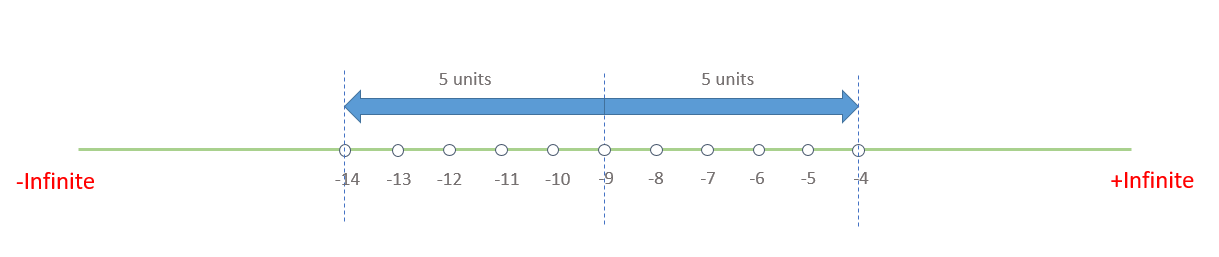 Thus, the values of x that satisfy the equation, |x + 9| = 5, are {14, -4}

o Coming to the second equation, where |x + 9| = 25, which can be written as, |x - (9) | = 25, the two numbers that are 25 units away from -9 are -34 and 16, as we can see in the diagram below.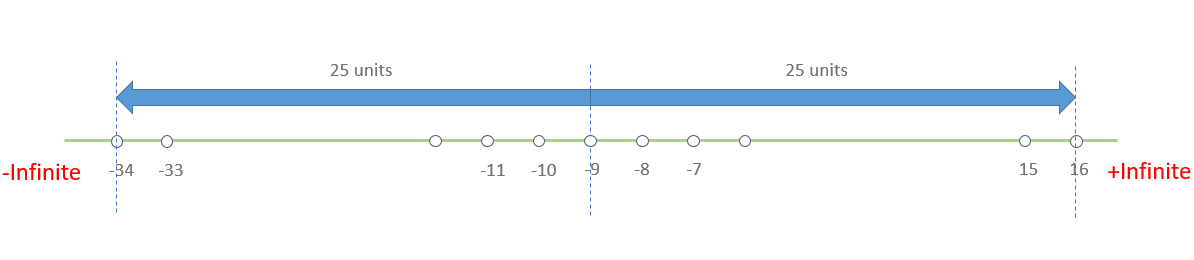 Thus, the values of x, that satisfy the equation, |x + 9| = 25, are {-34, 16}

Therefore, the values of x, that satisfy the equation, ||x + 9| - 15| = 10, are {-34, -14, -4, 16}

Key Takeaways

1. In this question, we learnt how to solve an absolute value equation involving more than 1 modulus.
a) And, to do that, we always start simplifying the equation from the outer most modulus and then we move to inner moduli.

2. We also learnt that substituting the inner moduli as a variable helps us to solve the question easily.

With these learnings in mind, let us now move to the next question.

Example 2

How many values of x, satisfy the equation ||2x - 7| - 3| = 12?
A. 0
B. 1
C. 2
D. 3
E. 4

Solution

Let's now use our learnings from previous question to solve an equation, which is pretty much similar to the previous equation except that the co-efficient of x is not equal to 1, in this question.

o So, as we already know, first, let's substitute the inner modulus, |2x - 7| as y
o Hence, we get, |y - 3| = 12

Now, applying the logic, that is shown in the concept video, we can say that y is a number, which is at a distance of 12 units from point, "3" on the number line
o Let's represent 3 on a number line and find the values of y that are 12 units away from "3"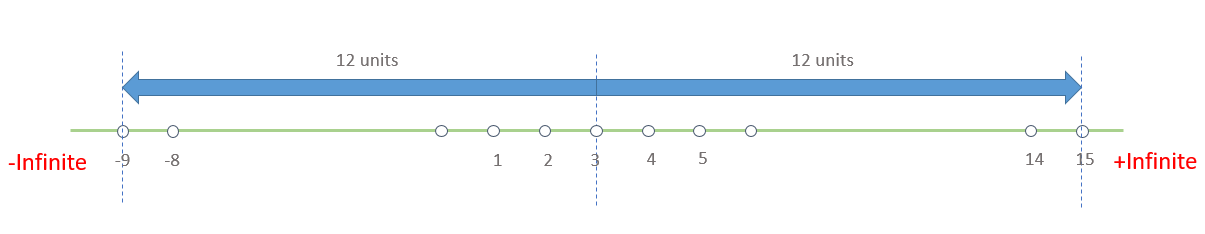So, from the above diagram, we can see that the two possible values of y are {-9, 15}

o Substituting back the modulus in the above equation, we get 2 equations,
o |2x - 7| = -9, or
o |2x - 7| = 15

Now, we need to solve each of the above two equations to find all the possible values of x.

o So, let's consider the first equation, |2x - 7| = -9. If we observe this equation carefully, we can say that this equation has no solution, since, the value of a modulus cannot be negative.
o Thus, we do not have to solve this equation. We can simply say that there is no value of x, which satisfies this equation.

Trap:
If you fail to notice this, you end up marking the answer as E, thinking, since, there are 2 moduli, we would be getting 4 values of x as seen in the previous question. But, then you will fall in the trap that the question maker has set for you.

So, we should always keep in mind the basic definition of absolute value while solving an equation.

o Now, considering the second equation, |2x - 7| = 15, we can solve this as the modulus value is greater than zero.

o So, as shown in the concept video, first we need to divide the equation by 2, on both sides, to make the coefficient of x as 1

o Dividing by 2, we get, |x - 3.5| = 7.5
o Now that, we have the equation in its general form, we can say that x is a number, which is at a distance of 7.5 units from the point "3.5"
o Representing this on a number line as shown below, we get the values of x as {-4, 11}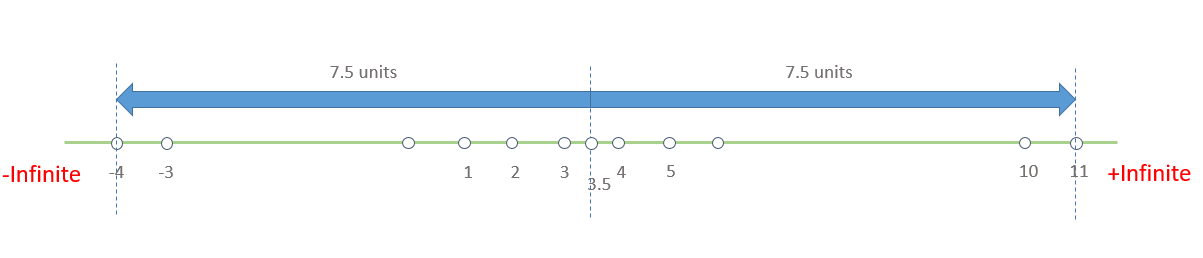o Therefore, the number of possible values of x that satisfies the given equation, ||2x - 7|-3| = 12, is 2

Hence the correct answer is Option C.

Key Takeaways

1. In this question, we learnt how to solve an absolute value equation in which the coefficient of x is not equal to 1.
a) To solve such equation, we need to divide the entire equation by the coefficient of x.
2. We have also learnt the importance of the basic condition of modulus, that is the value of a modulus cannot be negative, in finding the final answer

Next Topic - Absolute Inequalities

Now that you have mastered the concept of Absolute Equations, you have won half the battle in tackling Absolute value questions. Now, it's time to master the concept of Absolute Inequalities to win the other half.

o Click here to get started with the concept of Absolute Inequalities now.

Get amazing free resources

e-GMAT offers a variety of free video lessons (25+) and diagnostic quizzes (400+ questions) on different topics. Start your free trial and learn using our immersive course.

To score beyond Q49 on GMAT, it is very important that you master Algebra as it has significant application in other sections such as Number Properties, Word Problems and Geometry. In this article, we would help learn you a simple yet effective approach to solve questions on one of the most confusing topics in Algebra - Absolute Value equations. Then we share a link with you to learn Absolute Inequalities, so that you can solve any Absolute value question with ease.

We suggest that you follow the sequence mentioned below:

1. Get started by going through the video lessons embedded above to learn the basics.
2. Then go through the following questions in this article to learn how to solve Absolute Equation questions that involve multiple modulus.
3. Finally go through the concept of Absolute Inequalities, to continue learning.

Example 1

If x is an integer, then find the values of x that satisfy the equation ||x + 9| - 15| = 10?

Solution

As we can see that we are given one modulus inside another modulus:

o That is, |x+9| is inside ||x+9|-15|.
o And, we are asked to find out all the possible values of x such that ||x + 9| - 15| = 10.

So, how do we solve this question?

o Well, we will simply apply the basic properties of absolute values.

1. We will first solve the outermost modulus.

a. And, while solving it, we can substitute the inner modulus with an appropriate variable such as y.
2. Once we have solved the outer modulus, we will get the values for the assumed variable y and then we can simplify the inner modulus, by substituting back the modulus.
3. Now, this process will continue even if we had more than 2 moduli in the question.
a. We will first solve the outermost and then we move from outermost modulus to the inner moduli.

So, let's apply the same process here.

o We will start from the outer modulus.
o Therefore, let us substitute the inner modulus, |x+9|= y.
 Hence, we get |y - 15| = 10.

o Now, let's focus our energy on solving the equation |y - 15| = 10
 From the concept video, we learnt that the form |x - a| = b represents numbers on the number line that are at a distance of 'b' units from 'a'
 Applying this logic, to the equation |y - 15| = 10, we can say that y is a number at a distance of 10 units from "15".

o So, let's find out all the numbers, which are at a distance of 10 units from the point '15', by representing it on a number line.o If we observe the diagram above, we can notice that there can be two possible numbers, which are at a distance of 10 units from 15.
 One on the left side of 15, which is 10 units away from it = 15 - 10 = 5 and
 One on the right side of 15, which is 10 units away from it = 15 + 10 = 25

o Hence, the possible values of y are 5 and 25

o Substituting back the modulus in the above equation, we get,
 |x + 9| = 5, or
 |x + 9| = 25

o Let's solve each modulus individually and find the values of x

o Considering the first case, where |x + 9| = 5, we need to find the values of x, which are at a distance of 5 units from -9.
 Now, most of you might wonder, why did we consider -9, instead of 9. But, if we observe the general equation of modulus carefully, it is of the form |x - a| = b., where x is b units away from point 'a'.
 And, in this case, we can write |x + 9| = 5 as |x - (-9) | = 5.

Note: So, remember to notice the sign of 'a' carefully as it is a common mistake done by users and you would find one of the answer choices to set you up in this trap.

 So, representing -9 on a number line, we can find that the numbers, which are at a distance of 5 units from -9 are -14 and -4 Thus, the values of x that satisfy the equation, |x + 9| = 5, are {14, -4}

o Coming to the second equation, where |x + 9| = 25, which can be written as, |x - (9) | = 25, the two numbers that are 25 units away from -9 are -34 and 16, as we can see in the diagram below. Thus, the values of x, that satisfy the equation, |x + 9| = 25, are {-34, 16}

Therefore, the values of x, that satisfy the equation, ||x + 9| - 15| = 10, are {-34, -14, -4, 16}

Key Takeaways

1. In this question, we learnt how to solve an absolute value equation involving more than 1 modulus.
a) And, to do that, we always start simplifying the equation from the outer most modulus and then we move to inner moduli.

2. We also learnt that substituting the inner moduli as a variable helps us to solve the question easily.

With these learnings in mind, let us now move to the next question.

Example 2

How many values of x, satisfy the equation ||2x - 7| - 3| = 12?
A. 0
B. 1
C. 2
D. 3
E. 4

Solution

Let's now use our learnings from previous question to solve an equation, which is pretty much similar to the previous equation except that the co-efficient of x is not equal to 1, in this question.

o So, as we already know, first, let's substitute the inner modulus, |2x - 7| as y
o Hence, we get, |y - 3| = 12

Now, applying the logic, that is shown in the concept video, we can say that y is a number, which is at a distance of 12 units from point, "3" on the number line
o Let's represent 3 on a number line and find the values of y that are 12 units away from "3"So, from the above diagram, we can see that the two possible values of y are {-9, 15}

o Substituting back the modulus in the above equation, we get 2 equations,
o |2x - 7| = -9, or
o |2x - 7| = 15

Now, we need to solve each of the above two equations to find all the possible values of x.

o So, let's consider the first equation, |2x - 7| = -9. If we observe this equation carefully, we can say that this equation has no solution, since, the value of a modulus cannot be negative.
o Thus, we do not have to solve this equation. We can simply say that there is no value of x, which satisfies this equation.

Trap:
If you fail to notice this, you end up marking the answer as E, thinking, since, there are 2 moduli, we would be getting 4 values of x as seen in the previous question. But, then you will fall in the trap that the question maker has set for you.

So, we should always keep in mind the basic definition of absolute value while solving an equation.

o Now, considering the second equation, |2x - 7| = 15, we can solve this as the modulus value is greater than zero.

o So, as shown in the concept video, first we need to divide the equation by 2, on both sides, to make the coefficient of x as 1

o Dividing by 2, we get, |x - 3.5| = 7.5
o Now that, we have the equation in its general form, we can say that x is a number, which is at a distance of 7.5 units from the point "3.5"
o Representing this on a number line as shown below, we get the values of x as {-4, 11}o Therefore, the number of possible values of x that satisfies the given equation, ||2x - 7|-3| = 12, is 2

Hence the correct answer is Option C.

Key Takeaways

1. In this question, we learnt how to solve an absolute value equation in which the coefficient of x is not equal to 1.
a) To solve such equation, we need to divide the entire equation by the coefficient of x.
2. We have also learnt the importance of the basic condition of modulus, that is the value of a modulus cannot be negative, in finding the final answer

Next Topic - Absolute Inequalities

Now that you have mastered the concept of Absolute Equations, you have won half the battle in tackling Absolute value questions. Now, it's time to master the concept of Absolute Inequalities to win the other half.

o Click here to get started with the concept of Absolute Inequalities now.

Get amazing free resources

e-GMAT offers a variety of free video lessons (25+) and diagnostic quizzes (400+ questions) on different topics. Start your free trial and learn using our immersive course.

_________________
InternB
Joined: 21 Dec 2018
Posts: 17
Location: India
GMAT 1: 650 Q44 V35GMAT 2: 660 Q48 V32WE: Project Management (Transportation)
Re: Tips and Tricks for Absolute Values  [#permalink]

### Show TagsRe: Tips and Tricks for Absolute Values   [#permalink] 11 Jul 2019, 09:56
Display posts from previous: Sort by

# Tips and Tricks for Absolute Values